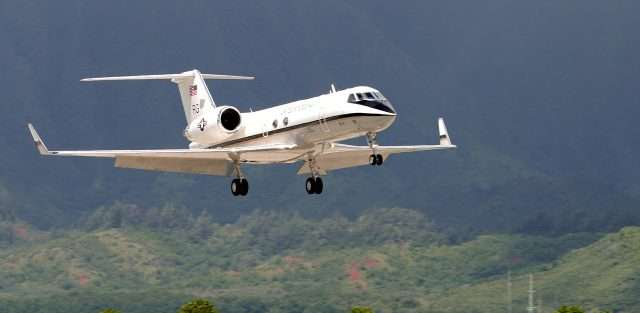# Standard Lapse RateIn aviation terms, standard lapse rate is the rate at which atmospheric temperature drops with an increase in altitude. The change can either be positive or negative, depending on the situation. However, the lapse rate will always decrease with height at a standard rate.

## Weather Plays a Part

Introducing moisture into the standard lapse rate equation can play a big role. If moisture is added, the laps rate will range from 2-3 degrees Fahrenheit per 1,000 feet. Assuming the weather conditions are cry, the lapse rate comes in at about 5.5 degrees Fahrenheit per 1,000 feet.

The standard lapse rate, in aviation terms, is calculated somewhere between the two. The standard lapse rate will typically decrease at a rate of roughly 3.5 degrees Fahrenheit/2 degrees Celsius per thousand feet, up to 36,000 feet. However, from 36,000 to 65,600 feet, temperatures are considered constant.

## A Pilot’s Job

Inversions, additions, and decreases in moisture will produce different lapse rates. Because the environmental conditions can affect the lapse rate and the standard lapse rate is only an average, pilots need to utilize constant pressure charts. These charts can help pilot’s determine how the atmosphere is actually behaving.

Temperatures and atmospheric conditions are important when drawing up flight plans. The best practice for pilots if to review all temperatures en route and consider the constant pressure charts as well.